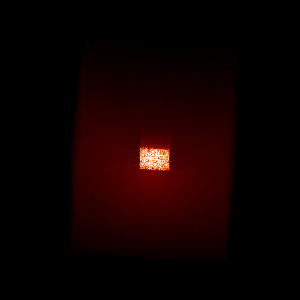# Making animations¶

In this tutorial, we will find out how to make animations from Hyperion output.

## Example model¶

To start with we will use a model very similar to Plotting and writing images but this time we only compute the image in one wavelength bin, but for a number of different viewing angles:

```import numpy as np

from hyperion.model import Model
from hyperion.util.constants import pc, lsun

# Initialize model
m = Model()

# Set up 64x64x64 cartesian grid
w = np.linspace(-pc, pc, 64)
m.set_cartesian_grid(w, w, w)

# Add density grid with constant density and add a higher density cube inside to
density = np.ones(m.grid.shape) * 1e-21
density[26:38, 26:38, 26:38] = 1.e-18

# Add a point source in the center
s.position = (0.4 * pc, 0., 0.)
s.luminosity = 1000 * lsun
s.temperature = 6000.

# Add multi-wavelength image for a single viewing angle
image.set_wavelength_range(1, 190., 210.)
image.set_viewing_angles(np.repeat(45., 36), np.linspace(5., 355., 36))
image.set_image_size(400, 400)
image.set_image_limits(-1.5 * pc, 1.5 * pc, -1.5 * pc, 1.5 * pc)

# Set runtime parameters. We turn off scattering for the imaging since it is not
# important at these wavelengths.
m.set_n_initial_iterations(5)
m.set_raytracing(True)
m.set_n_photons(initial=4e6, imaging=0,
raytracing_sources=1, raytracing_dust=1e7)

# Write out input file
m.write('flyaround_cube.rtin')
m.run('flyaround_cube.rtout', mpi=True)
```

Note

If you want to run this model you will need to download the `kmh_lite.hdf5` dust file into the same directory as the script above (disclaimer: do not use this dust file outside of these tutorials!).

## Making a fly-around movie¶

The following script describes how to generate PNG frames for an animation:

```import os

import numpy as np
import matplotlib.pyplot as plt

from hyperion.model import ModelOutput
from hyperion.util.constants import pc

# Create output directory if it does not already exist
if not os.path.exists('frames'):
os.mkdir('frames')

# Open model
m = ModelOutput('flyaround_cube.rtout')

image = m.get_image(distance=300 * pc, units='MJy/sr')

# image.val is now an array with four dimensions (n_view, n_y, n_x, n_wav)

for iview in range(image.val.shape):

# Open figure and create axes
fig = plt.figure(figsize=(3, 3))

# This is the command to show the image. The parameters vmin and vmax are
# the min and max levels for the grayscale (remove for default values).
# The colormap is set here to be a heat map. Other possible heat maps
# include plt.cm.gray (grayscale), plt.cm.gist_yarg (inverted grayscale),
# plt.cm.jet (default, colorful). The np.sqrt() is used to plot the
# images on a sqrt stretch.
ax.imshow(np.sqrt(image.val[iview, :, :, 0]), vmin=0, vmax=np.sqrt(2000.),
cmap=plt.cm.gist_heat, origin='lower')

# Save figure. The facecolor='black' and edgecolor='black' are for
# esthetics, and hide the axes
fig.savefig('frames/frame_%05i.png' % iview,
facecolor='black', edgecolor='black')

# Close figure
plt.close(fig)
```

The frames can then be combined into a GIF animation using ImageMagick:

```\$ convert -delay 10 -adjoin frames/*.png movie.gif
```

The delay value is the delay between frames in 1/100ths of a second. The result is the following: